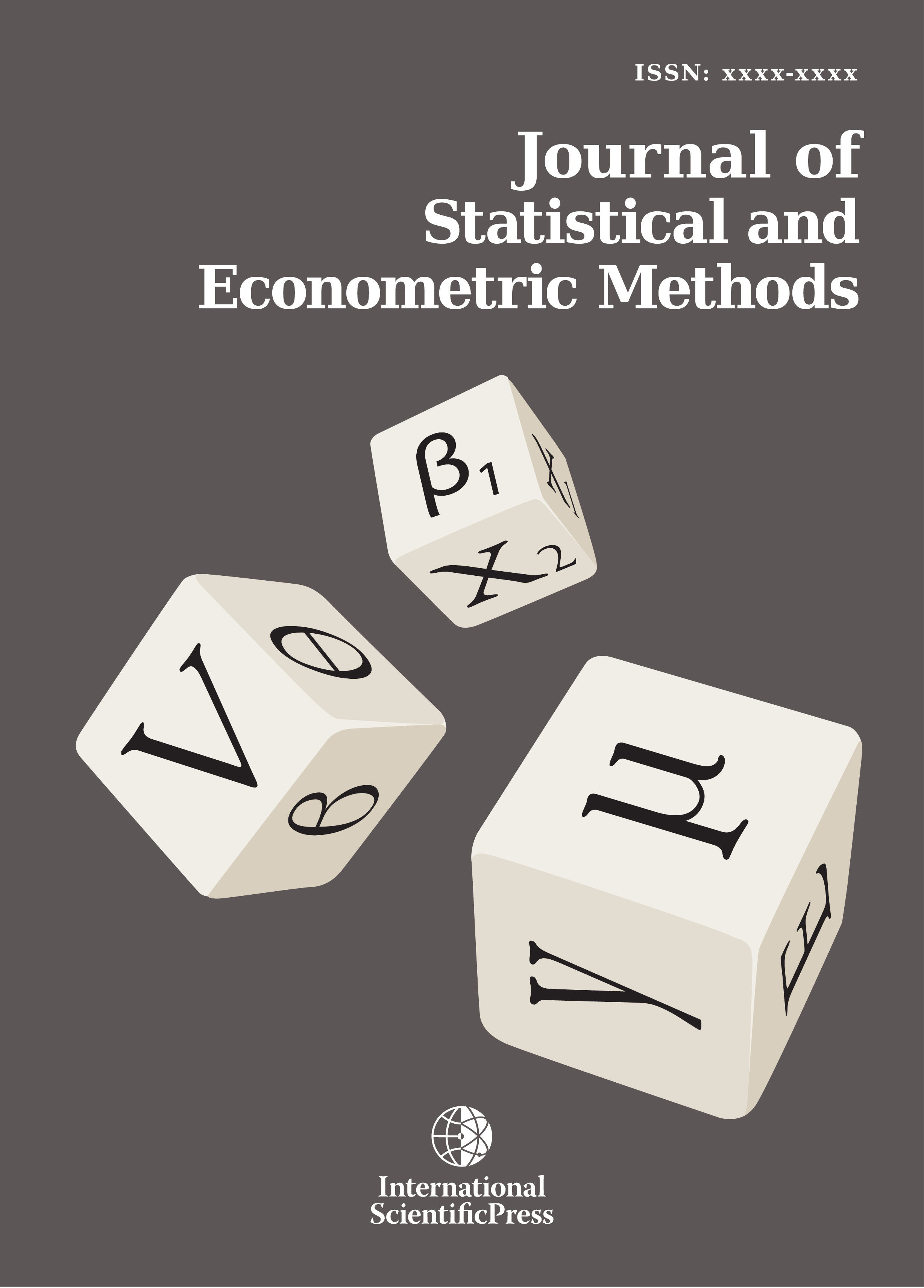# Journal of Statistical and Econometric Methods

#### Applications of Maximum Entropy Principle in Formulating Solution of Constrained Non-Linear Programming Problem

•[ Download ]
• Times downloaded: 1304
• Abstract

The maximum entropy principle finds its applications in a variety of disciplines related with Operations Research. The use of the principle can be extended to obtain the solution of a mathematical programming problem. In the present communication, an algorithm has been developed for solving constrained non-linear programming problem through the equivalent surrogate problem. In the solution process of so formed surrogate problem, the surrogate multipliers need to be updated and therefore, maximum entropy formulation has been used for the update of the multipliers. For the implementation and well understanding of the algorithm, an example has been discussed which confirms that entropy based updates for the surrogate multipliers lead to a fast and accurate solution to the problem.ISSN: 2241-0376 (Online)
2241-0384 (Print)